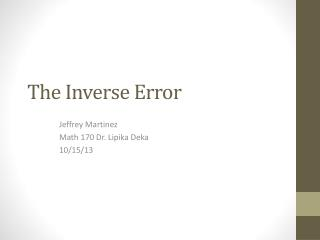DownloadDownload PresentationThe Inverse Error

# The Inverse Error

Download Presentation## The Inverse Error

- - - - - - - - - - - - - - - - - - - - - - - - - - - E N D - - - - - - - - - - - - - - - - - - - - - - - - - - -
##### Presentation Transcript

1. The Inverse Error Jeffrey Martinez Math 170 Dr. LipikaDeka 10/15/13

2. Inverse Error Also known as the fallacy of denying the antecedent (Epp p. 58). The inverse error is a reasoning error that an inexperienced logician may fall into, since in at least one case the conclusion can be true given the premises, but not in all cases. The premises present a given implication, and then state that the hypotheses, or antecedent is false. This step is called denying the antecedent. The conclusion then states that since the antecedent is not true, the consequent must not be true. Although at first glance the argument looks valid, but can be soon shown invalid by using truth tables, or by counterexample. We see an example of this error in exercise #29 in section 2.3 of our Discrete Mathematics and Applications textbook. We can see the problem on the next slide.

3. Section 2.3 #29 Use symbols to write the logical form of each argument. If the argument is valid, identify the rule of inference that guarantees its validity. Otherwise, state whether the converse or the inverse error is made. 29. Premise #1: If at least one of these two numbers is divisible by 6, then the product of these two numbers is divisible by 6. Premise #2: Neither of these two numbers is divisible by 6 ∴The product of these two numbers is not divisible by 6. Solution: Symbolically the initial implication (Premise #1) is of the basic form If R, then S, or R→S, letting R = At least one of these two numbers is divisible by 6. S = The product of these two numbers is divisible by 6. Premise #2 states that R is not true or ~R is true. The conclusion states that S is not true, or ~S is true. The simple answer is that the argument is invalid, because the inverse error is made. But lets look at truth table to prove this answer.

4. Looking at the truth table below, we identify our premises. We look for instances when both our premises R → S and ~R are true. In the fourth row, our premises are true and our conclusion ~S is true. But in the third row we see that even though R → S and ~R are true, ~S is not true. *R → S is only false when R is true, and S is false. This means that S could be true even when R is false, which is important to our solution. To state this as a counterexample, given the original implication “If at least one of these two numbers is divisible by 6, then the product of these two numbers is divisible by 6.” We let the two numbers be 2 and 3. Both 2 and 3 are NOT divisible by 6 (~R), but their product(2x3=6) IS divisible by 6 (S). Therefore the argument is invalid by counterexample. This is known as the inverse error, because ~R →~S is the inverse of our original implication. But we cannot use the inverse, because an implication and it’s inverse are not logically equivalent. Therefore, the conclusion is false, and the argument is invalid due to the inverse error.